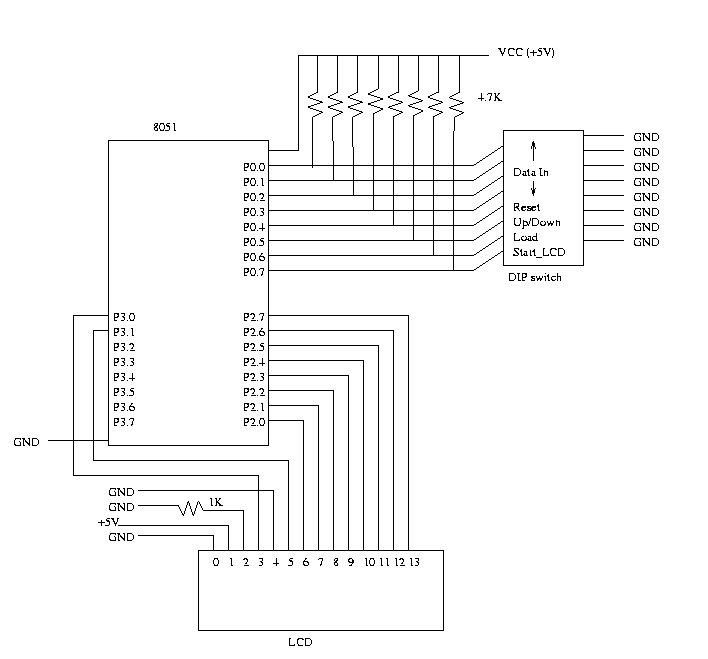Implementing a 4-bit counter using an 8051 and Interfacing it to an LCD

Introduction

In this lab, you will learn how to write a simple C program for 80X51 micro-controller, compile it using C51 compiler, and emulate it on an emulator using PDS51. The program will be used to control a simple 4-bit up-down counter, capable of counting from 0 to 15. At each step the count should be displayed in decimal format on the LCD.

Assignment

In this lab :

• You will design a 4-bit Up-Down counter using the C programming language for the 8051 micro-controller and display the count on an LCD.

• You will then test and run your program on the 8051.

• The 4 bit counter has the following functionality:
• The counter has the following input pins :
1. Reset : when high resets the counter dataout to ``0000''
2. Updown : decides whether the counter counts up or down.
3. Load : makes the counter count from the 4 bit input Datain
4. Datain : which is a 4 bit input count
• The counter has a 4 bit Dataout to reflect the count.
• The count has to be sent to the LCD and displayed in decimal format.

Apparatus Required

1. 4.7k resistors(8)
2. 1k resistor(1)
3. DIP switch
4. LCD
5. 5V power supply
6. Philips PDS51 development board

SchematicProgram

```
#pragma SMALL DB OE
#include <reg51.h>
#include "io.h"

/* P0, P1, P2 and P3 are predefined port names and are bit addressable */

sbit reset = P0^4; /* bit 4 of Port 0 */
sbit up_down = P0^5;
sbit Start_LCD = P0^7; /* bit 7 of Port 3 */

/* Delay function */
void delay() {

int i, j;

for(i=0; i<1000; i++)
for(j=0; j<100; j++)
i = i + 0;
}

/* Function to output the decimal value of the count on the LCD */
void PrintInt(unsigned char i) {
char ch;

/* Write code to convert the count to a string value and use the
PrintString function provided in io.c */

PrintString(ch);
}

void main(void) {

unsigned char count = 0;

InitIO();  /* Initialize the LCD */
while (1) {
if (Start_LCD == 1) {
ClearScreen();
delay();
}
else if (reset == 1) {

/* Output 0 on the LCD */

}
else if (load == 1) {

/* Output the current value of Datain on the LCD */

}
else {
/* Check the Up/Down pin for 1 or 0 count up or down
accordingly. Display each value on the LCD */
}
}
}

```

Procedure

1. Wire up the circuit as shown in the schematic.
Note: Port 0 should not be used for output because it does cannot sufficiently drive the LCD.
2. Map your network drive to
`           P:\\Peart\cs122`
3. Run the batch file cs122.bat
4. Get the IO files to control the LCD. The functions specified in these files are used to handle initialization and other special functions of the LCD.
5. Open up a DOS window and edit your program under C: For eg:
```	   C:\Temp\count.c
```
```	   c51 count.c
8. ```	   bl51 count.obj, io.obj to count.omf